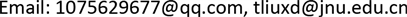﻿ 创业板公司财务指标与股价的关联性研究—基于制造业和信息技术业 The Correlation between Financial Indexes and Stock Prices in ChiNext—Based on Manufacturing and IT Industry

Statistics and Application
Vol.07 No.03(2018), Article ID:25381,10 pages
10.12677/SA.2018.73033

The Correlation between Financial Indexes and Stock Prices in ChiNext

—Based on Manufacturing and IT Industry

Meng Xu, Xiangdong Liu

College of Economics, Jinan University, Guangzhou GuangdongReceived: May 21st, 2018; accepted: Jun. 6th, 2018; published: Jun. 13th, 2018ABSTRACT

Most listed companies in GEM market of China have the characteristic of high risk and high income. The most common information that the investors can get about operating performance of listed companies is financial statements. Hence, it is necessary to study the relationship between the stock prices and financial indexes of the manufacturing and information technology companies in ChiNext. This article reduces the dimension of original financial indicators with factor analysis method, forming the profitability factor, operation capacity factor, debt paying ability factor and growth ability factor. The paper takes the four factors as independent variables, and takes stock prices as the dependent variable to establish the regression equation, discussing the specific relationship among all kinds of financial indicators and stock prices. It aims to provide a better reference for the investors to choose stocks and for the listed companies to improve their management ability. The empirical results show that the listed companies’ share prices in manufacturing industry have significant positive relationship with profitability factor, growth ability factor and debt paying ability factor, and it has no significant correlation with operation ability factor. As for information technology companies, their share price has no significant relationship with debt paying ability factor, and it is strongly influenced by the other three factors and most susceptible to the growth ability factor. Investors should pay more attention to the profitability of the company when investing in the stocks of manufacturing industry, and should focus on the company’s growth ability when investing in information technology industry. Companies in both industries should pay more attention to their solvency and operational capacity.

Keywords:ChiNext, Financial Indexes, Share Prices, Factor Analysis

—基于制造业和信息技术业1. 引言

2. 研究框架

3. 财务指标与股价的相关关系

3.1. 制造业财务指标与股价的相关关系

3.2. 信息技术业财务指标与股价的相关关系Table 1. The correlation coefficient of manufacturing industry

4. 财务指标与股价关系的实证研究

4.1. 财务指标降维——因子分析

4.1.1. 制造业财务指标因子分析Table 2. The correlation coefficient of IT industryTable 3. The accumulated variance contribution ratio of manufacturing industry

1) 因子1在每股收益X1、净资产报酬率X2、主营业务利润率X3、成本费用利润率X4四个财务指标上有较大载荷，因此因子1为盈利能力因子。

2) 因子2在资产负债率X5、流动比率X6、速动比率X7上有较大载荷，因此因子2为偿债能力因子。

3) 因子3在净资产增长率X9与总资产增长率X10上有较大载荷，因此因子3为成长能力因子。

4) 因子4在应收账款周转率X11、存货周转率X12与总资产周转率X13上有较大载荷，因此因子4为营运能力因子。

$\begin{array}{l}{F}_{1}=0.259{x}_{1}+0.319{x}_{2}+0.309{x}_{3}+0.300{x}_{4}+0.078{x}_{5}-0.034{x}_{6}-0.084{x}_{7}\\ \text{\hspace{0.17em}}\text{\hspace{0.17em}}\text{\hspace{0.17em}}\text{\hspace{0.17em}}\text{\hspace{0.17em}}\text{\hspace{0.17em}}+0.096{x}_{8}-0.132{x}_{9}-0.052{x}_{10}+0.030{x}_{11}-0.098{x}_{12}-0.023{x}_{13}\end{array}$

$\begin{array}{l}{F}_{2}=-0.065{x}_{1}-0.137{x}_{2}+0.016{x}_{3}+0.034{x}_{4}-0.343{x}_{5}+0.333{x}_{6}+0.349{x}_{7}\\ \text{\hspace{0.17em}}\text{\hspace{0.17em}}\text{\hspace{0.17em}}\text{\hspace{0.17em}}\text{\hspace{0.17em}}\text{\hspace{0.17em}}-0.142{x}_{8}+0.137{x}_{9}+0.028{x}_{10}+0.085{x}_{11}+0.072{x}_{12}-0.022{x}_{13}\end{array}$

$\begin{array}{l}{F}_{3}=0.030{x}_{1}-0.062{x}_{2}-0.063{x}_{3}-0.078{x}_{4}-0.079{x}_{5}+0.029{x}_{6}+0.065{x}_{7}\\ \text{\hspace{0.17em}}\text{\hspace{0.17em}}\text{\hspace{0.17em}}\text{\hspace{0.17em}}\text{\hspace{0.17em}}\text{\hspace{0.17em}}+0.142{x}_{8}+0.532{x}_{9}+0.485{x}_{10}-0.070{x}_{11}+0.003{x}_{12}+0.009{x}_{13}\end{array}$

$\begin{array}{l}{F}_{4}=0.053{x}_{1}+0.080{x}_{2}-0.105{x}_{3}-0.121{x}_{4}-0.068{x}_{5}+0.051{x}_{6}+0.052{x}_{7}\\ \text{\hspace{0.17em}}\text{\hspace{0.17em}}\text{\hspace{0.17em}}\text{\hspace{0.17em}}\text{\hspace{0.17em}}\text{\hspace{0.17em}}-0.013{x}_{8}-0.002{x}_{9}-0.058{x}_{10}+0.348{x}_{117}+0.439{x}_{12}+0.454{x}_{13}\end{array}$Table 4. The rotation component matrix of manufacturing industry

4.1.2. 信息技术业财务指标因子分析

1) 因子1在每股收益X1、净资产报酬率X2、主营业务利润率X3、成本费用利润率X4四个财务指标上有较大载荷，因此因子1为盈利能力因子。Table 5. The matrix of factor score function in manufacturing industryTable 6. The accumulated variance contribution ratio of IT industryTable 7. The rotation component matrix of IT industry

2) 因子2在资产负债率X5和流动比率X6、速动比率X7上具有较高载荷，这两个指标可以衡量公司的偿债能力，因此因子2为偿债能力因子。

3) 因子3在主营业务增长率X8与净资产增长率X9上具有较高载荷，这两个因子同属于成长能力指标，因此因子3为成长能力因子。

4) 因子4对于总资产周转率X13的解释力度最大，因此因子4为营运能力因子。

$\begin{array}{l}{F}_{1}=0.259{x}_{1}+0.324{x}_{2}+0.307{x}_{3}+0.304{x}_{4}+0.054{x}_{5}-0.091{x}_{6}-0.075{x}_{7}\\ \text{\hspace{0.17em}}\text{\hspace{0.17em}}\text{\hspace{0.17em}}\text{\hspace{0.17em}}\text{\hspace{0.17em}}\text{\hspace{0.17em}}-0.053{x}_{8}-0.056{x}_{9}-0.023{x}_{10}-0.035{x}_{11}-0.034{x}_{12}-0.026{x}_{13}\end{array}$

$\begin{array}{l}{F}_{2}=-0.073{x}_{1}-0.148{x}_{2}-0.014{x}_{3}+0.010{x}_{4}-0.306{x}_{5}+0.366{x}_{6}+0.361{x}_{7}\\ \text{\hspace{0.17em}}\text{\hspace{0.17em}}\text{\hspace{0.17em}}\text{\hspace{0.17em}}\text{\hspace{0.17em}}\text{\hspace{0.17em}}-0.053{x}_{8}-0.056{x}_{9}-0.023{x}_{10}-0.035{x}_{11}-0.034{x}_{12}-0.026{x}_{13}\end{array}$

$\begin{array}{l}{F}_{3}=0.061{x}_{1}-0.035{x}_{2}-0.077{x}_{3}-0.085{x}_{4}+0.021{x}_{5}+0.044{x}_{6}+0.018{x}_{7}\\ \text{\hspace{0.17em}}\text{\hspace{0.17em}}\text{\hspace{0.17em}}\text{\hspace{0.17em}}\text{\hspace{0.17em}}\text{\hspace{0.17em}}+0.479{x}_{8}+0.499{x}_{9}-0.077{x}_{10}-0.093{x}_{11}+0.145{x}_{12}+0.029{x}_{13}\end{array}$

$\begin{array}{l}{F}_{4}=0.073{x}_{1}-0.074{x}_{2}-0.125{x}_{3}-0.128{x}_{4}+0.060{x}_{5}+0.074{x}_{6}+0.006{x}_{7}\\ \text{\hspace{0.17em}}\text{\hspace{0.17em}}\text{\hspace{0.17em}}\text{\hspace{0.17em}}\text{\hspace{0.17em}}\text{\hspace{0.17em}}-0.006{x}_{8}+0.004{x}_{9}+0.365{x}_{10}+0.283{x}_{117}+0.305{x}_{12}+0.428{x}_{13}\end{array}$

4.2. 公司财务指标与股价的回归分析

4.2.1. 制造业财务指标与股价的回归分析

1) 盈利能力因子、偿债能力因子、成长能力因子的系数均通过了显著性检验，且盈利能力因子的系数5.295是四个因子中系数最大的，表明盈利能力对股价的影响程度最大。

2) 营运能力因子系数的P值为0.408，远大于0.05，因而制造业公司股价不受营运能力影响。

3) 盈利能力因子、偿债能力因子、成长能力因子系数均大于0，说明这三种能力对公司股价有正向影响。

4.2.2. 信息技术业财务指标与股价的回归分析

1) 成长能力因子的系数最大，说明信息技术业公司的股价最容易受到公司成长能力的正向影响。

2) 盈利能力因子和营运能力因子对股价的影响仅次于成长能力因子之后，且对股价呈同向变化。

3) 偿债能力因子前的系数未通过显著性检验，说明偿债能力对公司的股价没有显著影响。Table 8. The matrix of factor score function in IT industryTable 9. The general test of multiple regression model in manufacturing industryTable 10. The coefficient of factors in regression model of manufacturing industryTable 11. The general test of multiple regression model in IT industryTable 12. The coefficient of factors in regression model of IT industry

5. 结论

1) 本文所提取的四个公共因子的线性组合对创业板市场制造业和信息技术行业公司股价的拟合程度都很低，表明这两个行业公司的股价更大程度上受到的是非财务因素的影响，而目前财务指标并不能很好地说明股票的内在价值。

2) 两个行业公司的股价与偿债能力指标的关系很弱，甚至是没有关系，表明这两个行业的投资者对公司的偿债能力不够重视。

3) 创业板市场制造业公司的股价与公司盈利能力和成长能力呈正相关，且最容易受到公司盈利能力的影响，成长能力仅次于其后，而与营运能力并无显著相关性。

4) 创业板信息技术业公司的股价受公司成长能力的影响最大，公司的成长能力越强，股价越高；其次就是最易受到盈利能力的影响，而偿债能力对股价并无显著影响。

The Correlation between Financial Indexes and Stock Prices in ChiNext—Based on Manufacturing and IT Industry[J]. 统计学与应用, 2018, 07(03): 281-290. https://doi.org/10.12677/SA.2018.73033

1. 1. 宫情情. 上市公司内部治理对公司绩效影响的实证研究[D]: [硕士学位论文]. 蚌埠: 安徽财经大学, 2014.

2. 2. 王淑哲. 财务治理结构与公司绩效关系的实证研究——基于创业板上市公司2011~2013年面板数据[D]: [硕士学位论文]. 济南: 山东财经大学, 2015.

3. 3. 洪伟. 我国上市公司股票价格与财务指标相关性研究[D]: [硕士学位论文]. 南昌: 江西财经大学, 2013.

4. 4. 张开睿. 节能环保类上市公司财务信息对股票价格的影响研究[D]: [硕士学位论文]. 哈尔滨: 哈尔滨工业大学, 2015.

5. 5. 于晴. 上市公司财务信息对股票价格的影响——基于医药制造业的实证研究[D]: [硕士学位论文]. 扬州: 扬州大学, 2016.

6. 6. Habib, A. (2010) Value Relevance of Alternative Accounting Performance Measures: Australia Evidence. Ac-counting Research Journal, 23, 191-212.

7. 7. Chaudhry, M.I. and Sam, A.G. (2014) The Information Content of Accounting Earnings, Book Values, Losses and Firms Size vis-à-vis Stocks: Empirical Evidence from an Emerging Stock Market. Applied Financial Economics, 24, 1515-1527. https://doi.org/10.1080/09603107.2014.925074

8. 8. 张媛媛. 我国创业板上市公司财务指标与股价收益率的关联性研究[D]: [硕士学位论文]. 上海: 华东政法大学, 2016.

9. 9. 苍玉权, 严华麟. 公司财务状况与股票收益[J]. 数理统计与管理, 2005, 24(3): 86-92.

10. 10. 于洋, 赵璟. 股价泡沫检验及财务指标与股价的相关性研究[J]. 财经纵横, 2017, 43(4): 170-172.